# Circuit measurement temperature application

Share
/ 2019-04-22 / News

Figure 1 shows the basic circuit for measuring temperature. In the figure, A&prime;, B&prime; is the thermocouple compensation wire, t0 is the cold junction temperature of the thermocouple after using the compensation wire. In actual use, the compensation wire is extended to The terminal of the measuring instrument, the cold end temperature is the ambient temperature at which the instrument terminal is located.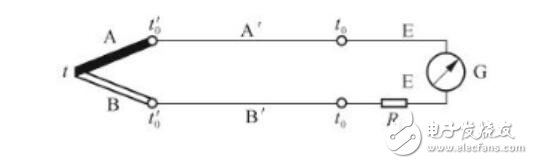Figure 1: Basic circuit for measuring temperature

As shown in 2, a plurality of measured temperatures are respectively measured by a plurality of thermocouples of the same type, and a plurality of thermocouples share one display instrument.They use the multiplexer to switch the temperature measurement point. The multi-point temperature measurement circuit is used in the automatic tour detection, and the temperature value of each measurement point is displayed as required, and only one display instrument and the compensation thermocouple are needed.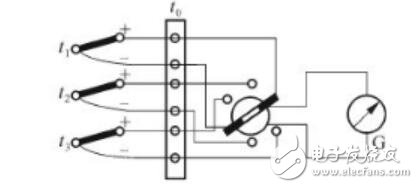Figure 2: Measuring multi-point temperature circuit

Figure 3 shows the temperature measurement circuit for measuring the temperature difference between two points. Two identical thermocouples are connected in series in reverse series with the same compensation wire. This connection method makes the meter measure the heat generated by two thermocouples. The difference in electromotive force, so the temperature difference between t1 and t2 can be measured.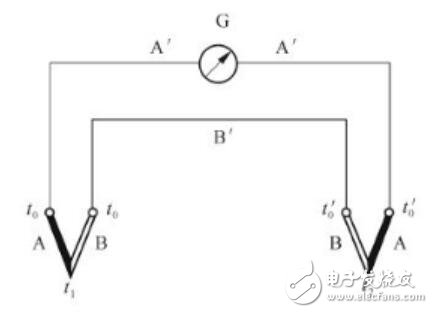Figure 3: Measuring the temperature difference circuit

Using a thermocouple The average temperature is measured by a parallel connection of thermocouples, as shown in Figure 4. Three thermocouples of the same type are connected in parallel, working in the linear part of the characteristic curve. Each thermocouple is connected in series with a larger equalizing resistor R. The millivolts input to the two ends of the meter are the sum of the thermoelectric potentials of the three thermocouples. That is, E = (E1 + E2 + E3) / 3, so it can reflect the average temperature of the three temperature measurement points. The average temperature of the measured area can be measured, and the meter is indexed the same as when using a thermocouple;The disadvantage is that when a thermocouple is still working properly after disconnection, the meter cannot be quickly reflected.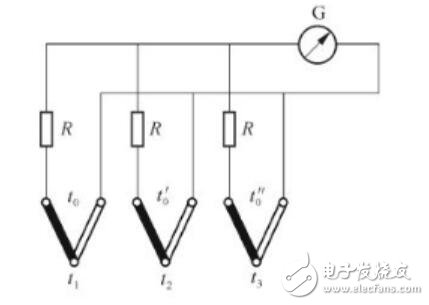Figure 4: Measuring Average Temperature Circuit

Using Thermocouple The method of measuring the temperature measurement circuit of several sums of temperatures generally adopts the same direction series connection of several thermocouples of the same type, as shown in FIG. 5, the sum of the thermoelectromotive forces input to both ends of the meter, that is, E=E1+E2+E3. The advantage of this type of circuit is that the thermoelectric potential disappears immediately when one thermocouple is disconnected, and the thermoelectric potential drops immediately when the other thermocouple is disconnected, and the meter can be immediately reflected.In addition, a large thermoelectromotive force can be obtained.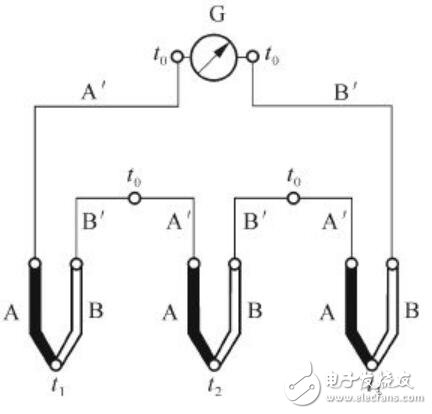Figure 5: Measuring Temperature and Circuitry

Relevant articles recommend

Top suppliers

Product:Photo Coupler,Optocoupler,IC,TOS,ON,AVAGO,SHARP,RENESAS,CT,EL,LTV,TI,NXP,ST
1YRS
Product:Infrared Receiver Module,Infrared Emitting Diode,Silicon PIN Photodiode,Silicon Phototransistor,LED,Silicon Photocell,Hall Effect IC,Photoelectric switch,Photoelectric encoder,IC.
1YRS
Product:KSD301 KSD9700 thermostat thermal protector fuse sensor
1YRS
Product:IC,PCB,module,capacitor,resistor,diodes,LED,LCD
1YRS
Product:Electronics components，ICs，MCU，RS-485/RS-422 Transceiver
9YRS
Subscribe News